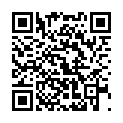# North Coast Synthesis Ltd.

## E♭m4+2+♯1Choose audio sample set:
MSK 007 Leapfrog VCF (patch; see in online store)
MSK 009 Coiler VCF (patch; see in online store)

Equivalent chord symbols: E♭m4+2+♭2, E♭m4+2+♭9, E3+2+♯1+♯7, E3+2+♯1+♭1, G♭2+6+7+♯7, G♭2+6+7+♭1.

Notes in this chord: E♭, E, F, G♭, A♭. Integer notation: {3, 4, 5, 6, 8}.

Nearby chords (one less note): E♭m4+2, E3+2+♯1, G♭2+6+7, E3+♯1+♯7, E♭2+♯1+♯2.

Nearby chords (one more note): EM9+♯1, A♭7+6+♯5, EM9♭5+♯1, D♭+2+4+♯2, E♭4+2+♯1+♯2, Ddim+2+♯1+♯3, E♭dim+2+4+♯1.

Parallel chords (same structure, different root): Cm4+2+♯1, Dm4+2+♯1, Em4+2+♯1, Fm4+2+♯1, Gm4+2+♯1, Am4+2+♯1, Bm4+2+♯1, D♭m4+2+♯1, G♭m4+2+♯1, A♭m4+2+♯1, B♭m4+2+♯1.

Experimental fretting charts for guitar standard EADGBE tuning (change tuning or instrument):